Courses

# NCERT Exemplar - Constructions Class 10 Notes | EduRev

## Class 10 : NCERT Exemplar - Constructions Class 10 Notes | EduRev

The document NCERT Exemplar - Constructions Class 10 Notes | EduRev is a part of the Class 10 Course Mathematics (Maths) Class 10.
All you need of Class 10 at this link: Class 10

Q.1. Draw a circle of radius 4 cm. Construct a pair of tangents to it, the angle between which is 60°. Also justify the construction. Measure the distance between the centre of the circle and the point of intersection of tangents.
Ans.
Steps of Construction:
Step I: Draw a circle with centre O and radius 4 cm.
Step II: Draw any diameter AOB.
Step III: Draw a radius OC such that ∠BOC = 60°.
Step IV: At C, we draw CM ⊥ OC and at A, we draw AN ⊥ OA.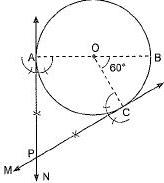Step V: Let the two perpendiculars intersect each other at P.
Then, PA and PC are required tangents.
Justification:
Since OA is the radius, so PA has to be a tangent to the circle. Similarly, PC is also tangent to the circle.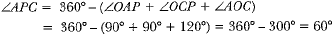Hence, tangents PA and PC are inclined to each other at an angle of 60°.

Q.2. Draw a triangle ABC in which AB = 4 cm, BC = 6 cm and AC = 9 cm. Construct a triangle similar to ΔABC with scale factor 3/2. Justify the construction. Are the two triangles congruent? Note that all the three angles and two sides of the two triangles are equal.
Ans.
Steps of Construction
Step I: Draw a line segment BC = 6 cm.
Step II: With centre B and radius 4 cm draw an arc.
Step III: With centre C and radius 9 cm draw another arc which intersects the previous arc at A.
Step IV: Join BA and CA. ABC is the required triangle.
Step V: Through B, draw an acute angle CBX on the side opposite to vertex A.
Step VI: Locate three arcs B1, B2 and B3 on BX such that BB1 - B1B2 = B2B3.
Step VII: Join B2C.
Step VIII: Draw B3C' || B2C intersecting the extended line segment BC at C’.
Step IX: Draw C'A' || CA intersecting the extended line segment BA at A'.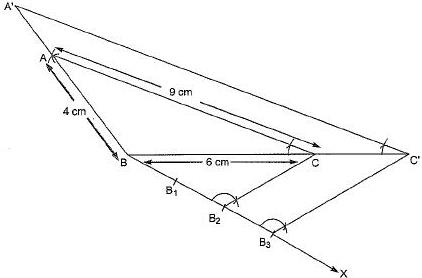Thus, ΔA'BC' is the required triangle (ΔA'BC' - ΔABC).
The two triangles are not congruent because, if two triangles are congruent, then they have same shape and size. Here, all tree angles are equal but all three sides are not equal.
Justification:
∵ B2C || B3C'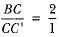Now,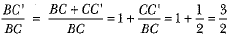Again, CC' || AC
ΔABC - ΔA'BC'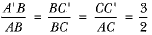Q.3. Two line segments AB and AC include an angle of 60° where AB = 5 cm and AC = 7 cm. Locate points P and Q on AB and AC, respectively such that AP = 3/4 AB and AQ = 1/4 AC. Join P and Q and measure the length PQ.
Ans.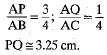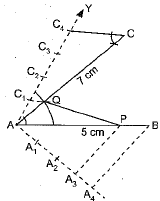Q.4. Draw a parallelogram ABCD in which BC = 5 cm, AB = 3 cm and ∠ABC = 60°, divide it into triangles BCD and ABD by the diagonal BD. Construct the triangle BD'C' similar to ΔBDC with scale factor 4/3. Draw the line segment D'A' parallel to DA where A' lies on extended side BA. Is A'BC'D a parallelogram?
Ans.
Yes, A'BC'D' is required || gm.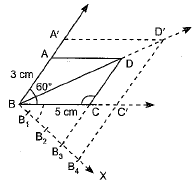Q.5. Draw an isosceles triangle ABC in which AB = AC = 6 cm and BC = 5 cm. Construct a triangle PQR similar to ΔABC in which PQ = 8 cm. Also justify the construction.
Ans.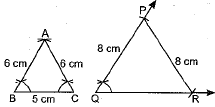In ΔPQR
∠Q = ∠B, PQ = PR = 8 cm.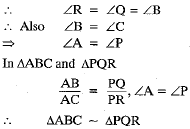Offer running on EduRev: Apply code STAYHOME200 to get INR 200 off on our premium plan EduRev Infinity!

## Mathematics (Maths) Class 10

62 videos|363 docs|103 tests

,

,

,

,

,

,

,

,

,

,

,

,

,

,

,

,

,

,

,

,

,

;